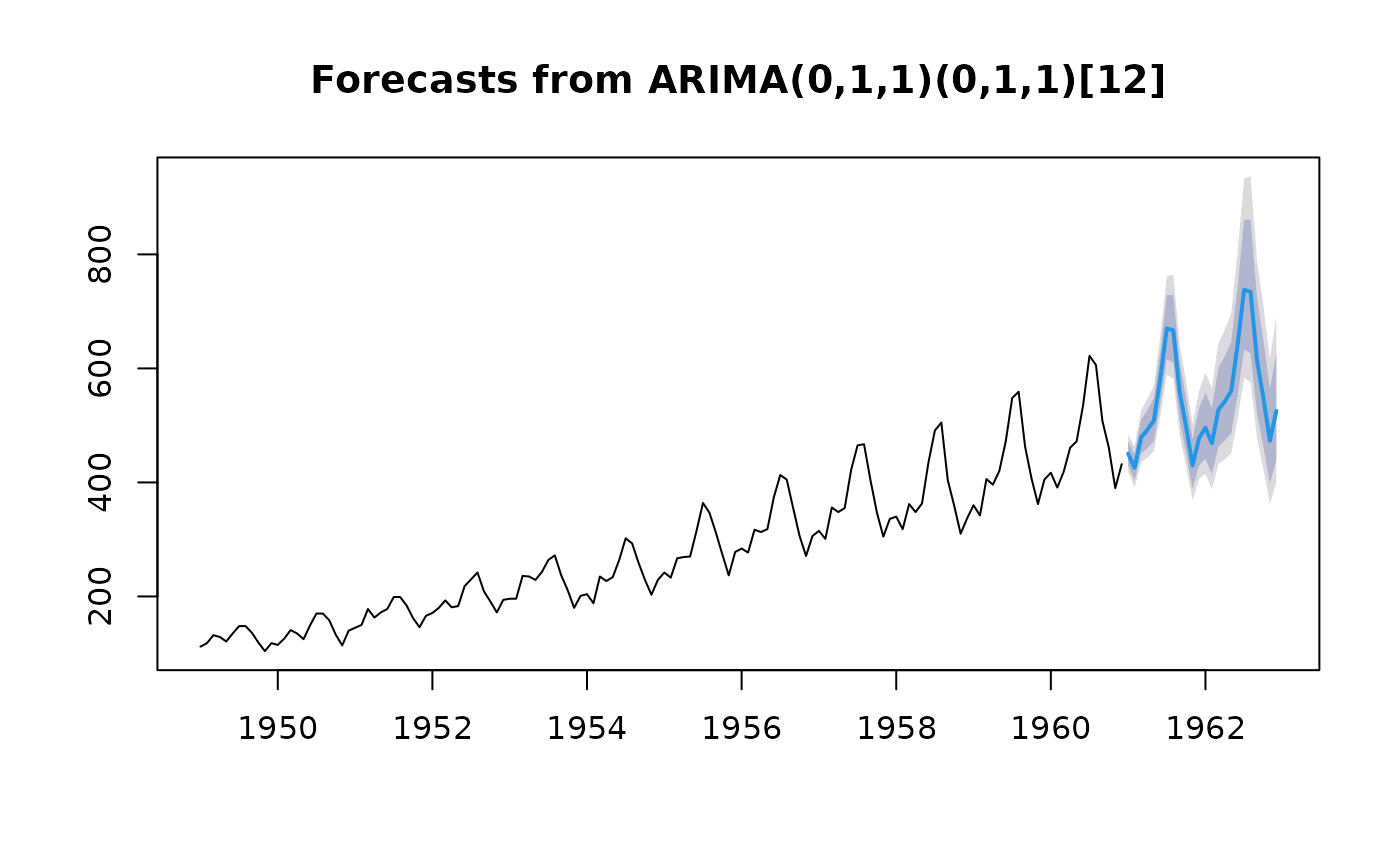If method=="guerrero", Guerrero's (1993) method is used, where lambda minimizes the coefficient of variation for subseries of x.

BoxCox.lambda(x, method = c("guerrero", "loglik"), lower = -1, upper = 2)

## Arguments

x

a numeric vector or time series of class ts

method

Choose method to be used in calculating lambda.

lower

Lower limit for possible lambda values.

upper

Upper limit for possible lambda values.

## Value

a number indicating the Box-Cox transformation parameter.

## Details

If method=="loglik", the value of lambda is chosen to maximize the profile log likelihood of a linear model fitted to x. For non-seasonal data, a linear time trend is fitted while for seasonal data, a linear time trend with seasonal dummy variables is used.

BoxCox

## Author

Leanne Chhay and Rob J Hyndman

## Examples


lambda <- BoxCox.lambda(AirPassengers,lower=0)
air.fit <- Arima(AirPassengers, order=c(0,1,1),
seasonal=list(order=c(0,1,1),period=12), lambda=lambda)
plot(forecast(air.fit))